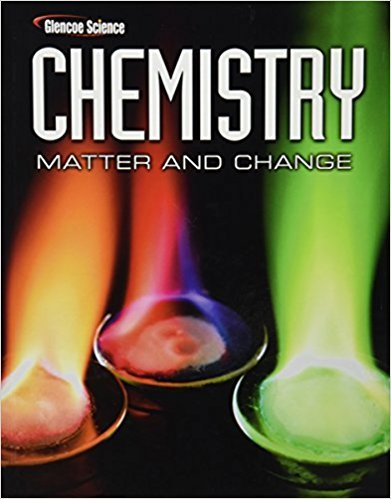×
×

# Solutions for Chapter 13: Gases## Full solutions for Chemistry: Matter & Change | 1st Edition

ISBN: 9780078746376Solutions for Chapter 13: Gases

Solutions for Chapter 13
4 5 0 401 Reviews
26
0
##### ISBN: 9780078746376

This textbook survival guide was created for the textbook: Chemistry: Matter & Change, edition: 1. This expansive textbook survival guide covers the following chapters and their solutions. Chapter 13: Gases includes 119 full step-by-step solutions. Chemistry: Matter & Change was written by and is associated to the ISBN: 9780078746376. Since 119 problems in chapter 13: Gases have been answered, more than 72546 students have viewed full step-by-step solutions from this chapter.

Key Chemistry Terms and definitions covered in this textbook
• Amino group

A compound containing an sp3 -hybridized nitrogen atom bonded to one, two, or three carbon atoms

• amphiprotic

Refers to the capacity of a substance to either add or lose a proton 1H+2. (Section 16.2)

• Celsius scale

A temperature scale on which water freezes at 0° and boils at 100° at sea level. (Section 1.4)

• Claisen rearrangement

A [3,3] sigmatropic rearrangement that is observed for allylic vinylic ethers.

• degenerate

A situation in which two or more orbitals have the same energy. (Section 6.7)

• electron configuration

The arrangement of electrons in the orbitals of an atom or molecule (Section 6.8)

• electron density

The probability of finding an electron at any particular point in an atom; this probability is equal to c2, the square of the wave function. Also called the probability density. (Section 6.5)

• Energy diagram

A graph showing the changes in energy that occur during a chemical reaction; energy is plotted on the vertical axis, and reaction progress is plotted on the horizontal axis. Also called a reaction coordinate diagram

• Equivalent hydrogens

Hydrogens that have the same chemical environment

• Imide

A functional group in which two acyl groups, RCO! or ArCO!, are bonded to a nitrogen atom

• Imine

A compound containing a carbon-nitrogen double bond, R2C"NR’; also called a Schiff base

• initiation

• Kiliani-Fischer synthesis

A process by which the chain of a carbohydrate is lengthened by one carbon atom.

• l-Monosaccharide

A monosaccharide that, when written as a Fischer projection, has the !OH on its penultimate carbon to the left.

• Lewis symbol (electron-dot symbol)

The chemical symbol for an element, with a dot for each valence electron. (Section 8.1)

• molecule

A chemical combination of two or more atoms. (Sections 1.1 and 2.6)

• momentum

The product of the mass, m, and velocity, v, of an object. (Section 6.4)

• optically inactive

A compound that does not rotate plane-polarized light.

• Protic solvent

A solvent that is a hydrogen-bond donor. Common protic solvents are water, low-molecular-weight alcohols, and low-molecular weight carboxylic acids.

• zwitterion

A net neutral compound that exhibits charge separation. Amino acids exist as zwitterions at physiological pH.

×